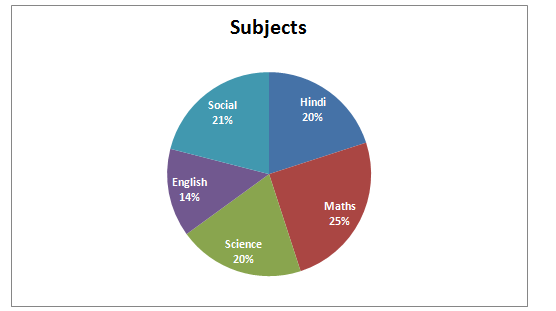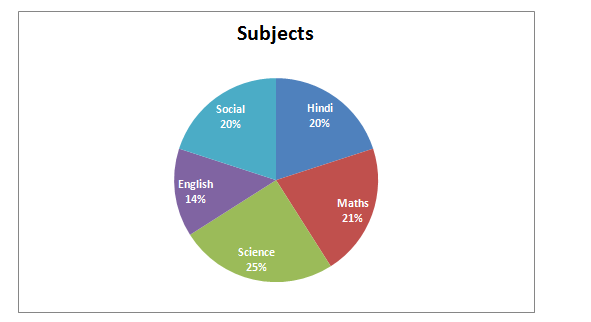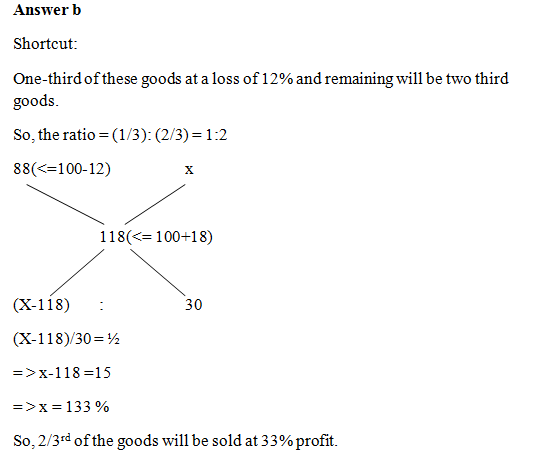# “20-20” Quantitative Aptitude | Crack SBI Clerk 2018 Day-38

Dear Friends, SBI Clerk 2018 Notification has been released we hope you all have started your preparation. Here we have started New Series of Practice Materials specially for SBI Clerk 2018. Aspirants those who are preparing for the exams can use this “20-20” Quantitative Aptitude Questions.

## Daily Practice Test Schedule | Good Luck

 Topic Daily Publishing Time Daily News Papers & Editorials 8.00 AM Current Affairs Quiz 9.00 AM Logical Reasoning 10.00 AM Quantitative Aptitude “20-20” 11.00 AM Vocabulary (Based on The Hindu) 12.00 PM Static GK Quiz 1.00 PM English Language “20-20” 2.00 PM Banking Awareness Quiz 3.00 PM Reasoning Puzzles & Seating 4.00 PM Daily Current Affairs Updates 5.00 PM Data Interpretation / Application Sums (Topic Wise) 6.00 PM Reasoning Ability “20-20” 7.00 PM English Language (New Pattern Questions) 8.00 PM General / Financial Awareness Quiz 9.00 PM

[WpProQuiz 1437]

Click “Start Quiz” to attend these Questions and view Explanation

### Click Here for SBI Clerk Prelims and Mains 2018 – Full Length Mock Test

1. Rajesh takes a loan of 10000 and pays back Rs. 12597.12 after 3 years at some rate of interest compounded annually. Find the rate of interest.
1. 7%
2. 9%
3. 8%
4. 5%
5. None of these
1. Sri gave 50% of the amount he had to Jothi. Jothi gave 2/5th of what he received from sri to saratha. After paying Rs. 200 to the taxi driver out of the amount he gets from jothi, saratha is now left with Rs. 700. How much amount did Sri have?
1. 6500
2. 5000
3. 7000
4. 4500
5. None of these
1. A merchant mixes 2 types of tea, one costing Rs. 140 per kg and the other Rs. 160 per kg in the ratio of 3:4. If he sells the mixture at Rs. 170 per kg, find the approximate % profit/Loss.
1. 12 %
2. 15%
3. 21%
4. 24%
5. None of these
1. If Vasu purchased some goods for Rs. 1050, and sold one-third of these goods at a loss of 12% then at what gain % should the remaining goods be sold to gain 18% on the whole transaction?
1. 18 %
2. 33 %
3. 27 %
4. 25 %
5. None of these
1. Mahesh can do a work in 24 days. He works alone for 4 days and Naren alone finishes the remaining work in 25 days. Both of them together can complete the work in?
1. 50/ 7 days
2. 15 days
3. 18 days
4. 40/3 days
5. 60/7 days

Directions (Q. 6-10): What should come in place of question mark (?) in the following number series?

1. 172, 172, 147, 247, 22, ?
1. 345
2. 476
3. 422
4. 376
5. None of these
1. 272, 265, 251, 230, ?
1. 202
2. 174
3. 156
4. 215
5. None of these
1. 2, 6, 21, 79, 341, ?
1. 856
2. 1741
3. 937
4. 1346
5. None of these
1. 48, 60, 76, 100, 136, ?
1. 174
2. 225
3. 336
4. 188
5. None of these
1. 27, 55, 120, 246, 463, ?
1. 932
2. 1056
3. 807
4. 735
5. None of these

Directions (Q. 11-15): What approximate value should come in place of question mark (?) in the following questions?

1. (√321 × 25) ÷ 30 =? – 523.01
1. 538
2. 606
3. 710
4. 800
5. 434
1. 49.21 × 19.89 – 24.98 × 14. 12 = (36+?) × 9
1. 34
2. 43
3. 52
4. 67
5. 49
1. (26.112 – 16.732) / ? = 21
1. 21
2. 28
3. 9
4. 18
5. 31
1. 35% of 1579 + 29% of 4516 =?*41 + 468 + 773. 89 – 199. 78
1. 35
2. 20
3. 30
4. 25
5. 45
1. 5 1/5 of 189.75 + 6 2/7 of 231. 49 =? % of 498 + 856.85
1. 432
2. 118
3. 317
4. 234
5. 178

Directions (Q. 16 – 20): Study the following pie chart carefully to answer the questions below:

Percentage of students passed in different subjects (Total student = 8000)Percentage of girls passed in these subjects out of the total students. (Total number of girls = 5000)16). Find the pass percentage of boys in English?

1. 36
2. 37.5
3. 38
4. 37
5. 36.5

17). How many boys are passed in Science and Social together?

1. 1045
2. 1050
3. 1060
4. 1030
5. 1020

18). Find the average number of girls passed in Hindi, Maths and Science together?

1. 2050
2. 2150
3. 1100
4. 2000
5. 1550

19). Number of boys passed in Hindi is what per cent less than the number of Girls passed in Science?

1. 16
2. 14
3. 40
4. 52
5. 62

20). What is the respective ratio of number of boys passed in English to the number of boys passed in Social?

1. 21:34
2. 34:21
3. 31:24
4. 24:31

e. None of these

Amount = P(1+(r/100))n

12597.12 = 10000(1+(r/100))3

((100+r)/100)3 = 12597.12/10000

((100+r)/100)3 = 1259712/1000000

((100+r)/100)3 = (108/100)3

((100+r)/100) = 108/100

100+r =108

R= 8%

Let Sri’s amount be x,

Saratha now having the amount of 700,

=>(x*(50/100)*(2/5)) – 200 = 700

= > x*(50/100)*(2/5) = 900

= > x= 900*5 = 4500

Sri initially having an amount of Rs. 4500

Total kg’s of 2 types of tea is not given. But the ratio will be 3:4. Take that as, 3x and 4x

Cost price = 3x*140 + 4x*160 = 1060x

Selling price = 7x*170 = 1190x

Profit % = (130x/1060x)*100 = 1300/106

Profit % = 12.26 % = 12% (approx)

1.Mahesh one day work = 1/24

4 days work = 4/24 = 1/6

Remaining work = 1-(1/6) = 5/6

Naren alone finishes the remaining work in 25 days.

= > (5/6) * Naren’s whole work = 25

= > Naren’s whole work = 30 days

Mahesh and Naren’s one day work,

= > (1/24) + (1/30)

= > 3/40

Both Mahesh and Naren’s together complete the work in,

= > 40/3 days

Directions (Q. 6-10):

The pattern is, +02, -52, +102, -152, +202

The pattern is, -(1*7), -(2*7), -(3*7), -(4*7),..

The pattern is, *1+22, *2+32, *3+ 42, *4+ 52, *5+ 62,..

The difference of difference is, 4, 8, 12, 16…

The difference is, 33 +1, 43 +1, 53 +1, 63 +1, 73 +1

Directions (Q. 11-15):

(18 × 25)/30 = x – 523

15 = x – 523

X= 15+523 = 538

49 × 20 – 25 × 14 = (36 + x) × 9

980 – 350 = 324 +9x

9x = 980 – 350 – 324

9x = 306

X = 34

(262 – 172)/ ? = 21

676 – 289)/x = 21

387/x = 21

X = 387/21 = 18.42

X=18

35 × 16 + 29 × 45 = 41x +468 + 774 -200

560 + 1305 -468 -774+200 = 41x

823 = 41x

X = 823/41

X = 20

(26/5)*190 + (44/7)* 231 = 5x +857

988 + 1452 – 857 = 5x

1583 = 5x

X = 1583/5 = 317

Directions (Q. 16 – 20):

Total students for English = 0.14*8000=1120

Total girls passed in English=0.14*5000=700

Total boys passed in English=1120-700=420

Boys pass %= (420/1120)*100= 37.5%

Total Boys passed in Science = [(0.20*8000)-(0.25*5000)] =350

Total Boys passed in Social = [(0.21*8000)-(0.20*5000)] =680

Total boys are passed in Science and Social = 350+680 = 1030

Total number of girls passed in Hindi, Maths and Science =5000*(66/100)

Average no of girls passed in Hindi, Maths and Science= 5000*(66/100)*(1/3)

= >1100

Total number of boys passed in Hindi = [(0.20*8000)-(0.20*5000)]= 600

Total number of Girls passed in Science = [0.25*5000]= 1250

Required % = [(1250-600)/1250]*100 = 52%

Total boys passed in English = (0.14*8000)-(0.14*500)=420

Total Boys passed in Social = [(0.21*8000)-(0.20*5000)] =680

Ratio = 420:680 = 21:34